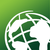# Create Random Points: distance, number of points

852
2
02-07-2014 10:59 AMNew Contributor
I am using the tool Create Random Points to create points with a mininum distance of 150m within an area. The constraining feature is a point layers consisting of points every 10 meters (center point of a MGRS grid). I have a question and have been experiencing a bug.

1) It seem mandatory to mention a number of points value. My goal is to create (select really) the maximum number of points that can fit within the area respecting the minimum distance. Not knowing what that number would be, I have put a number of points value exceeding what I think can fit. Is the tool going to fit in as many points as possible?

2) I have mentioned a minimum distance of 150m under the linear unit option. The points created are however sometimes closer than 150 m (110m, 130m, etc.). Any reason why that would be?

Tags (3)
2 RepliesOccasional Contributor
Hi Josee,

I think this article will help you understand how Create Random Points works. And to answer your first question, since your constraining feature is a point feature class, random points will be created at point feature locations also. So if the value you set exceeds the total number of points from the constraining point feature, it would just make sense that this value you inputted will not be honored. Please correct me if I'm wrong with this matter.New Contributor
Thank you for the article about how the points are randomly selected. It was very useful.

Following on 1). The number of points value I mentioned in the tool was always higher than could fit in my study area (about 30-50 points could fit) but lower than the number of points in the constraining feature layer (5300 points). The article you mentioned I believe answered my question in that "This process is repeated until the specified number of points is reached". In this case, since the number of point values cannot be reached but no more points can be added, the process would just stop and thus the maximum number of points that can fit would indeed be selected (I think).  Running the tool does result in a warning ("The specified number of points could not be created in all cases due to restrictions from the minimum allowed distance") but the tool still runs.

Following on 2).  Found the solution! I changed the projection of the input layer to UTMs which uses meters (instead of the decimal degree it used to be). Et voila! The minimum distance in meter is now respected.

As a side thought, this is the second time within the same project that changing the projection of the input layer results in the tool working properly (had problems going from UTMs to MGRS in the Convert Coordinate Notation tool). If specific projections are needed for the functioning of a tool, one would think the information would be mentioned in the instructions (or help menu) or the tool fixed? Anyway, things are working now.

Thanks!